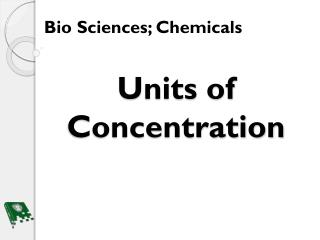Download PresentationUnits of Concentration# Units of Concentration - PowerPoint PPT Presentation

Download Presentation##### Units of Concentration

Download Policy: Content on the Website is provided to you AS IS for your information and personal use and may not be sold / licensed / shared on other websites without getting consent from its author. While downloading, if for some reason you are not able to download a presentation, the publisher may have deleted the file from their server.

- - - - - - - - - - - - - - - - - - - - - - - - - - - E N D - - - - - - - - - - - - - - - - - - - - - - - - - - -
##### Presentation Transcript

1. Units of Concentration Bio Sciences; Chemicals

2. Bellwork What is a homogeneous solution? A homogeneous solution has the same uniform appearance and composition throughout, specifically only one phase present.

3. Objectives 1. Recall the parts of a solution 2. Explain what concentration means 3. Give example of where concentration units are used 4. List common concentration units used in a lab 5. Differentiate between the various concentration units

4. Terminology Concentration: The amount of substance in a defined space or volume Percent w/w: Grams of solute divided by ml of total solution Percent w/v: Milliliters of solute divided by ml of total solution Percent v/v: Grams of solute divided by grams of total solution Molarity: Mole of solute divided by liters of solution Molality: moles of solute divided by kilograms of solvent Normality: Is a multiple of molarity or a gram equivalent weight of a solute per liter of solution Parts per Million: One milligram (mg) per liter of water or kilogram of something. Parts per Billion: One micro gram ( g) per liter of water or kilogram of something.

5. Solutions Recap What are the components of a solution Solvent Solute

6. Concentration Concentration: The amount of substance in a defined space or volume Defined space or volume Amount of substance

7. Where Are Concentration Units Where might you find concentration units; I need at least three (3) examples?

8. Common Concentration Units: Percent Percent (%). The mass/ volume of one components divided by the total mass/ volume w/v: Grams of solute divided by ml of total solution v/v: Milliliters of solute divided by ml of total solution w/w: Grams of solute divided by grams of total solution

9. Common Concentration Units: Molarity Specific number of particles

10. Common Concentration Units: Molarity cont. What is the molarity of a 100ml solution made from 3.0g of NaOH?

11. Common Concentration Units: Molality

12. Compare What is a similarity and a difference between Molarity and Molality?

13. Common Concentration Units: Normality Normality (N): Is a multiple of molarity either 1, 2, 3, 4, 5, etc. Or a gram equivalent weight of a solute per liter of solution Take 1N HCl: 1N means 1 molecule of H+ of Cl- per molecule of HCl Take 2N H2SO4: 2N means 2 molecules of H+ per molecule of H2SO4

14. Common Concentration Units: Parts Per Million and Billion

15. Closure Letter or Note to a Friend Write a letter or a note to a friend explaining the difference between three (3) types of the concentration units we learned in the lesson.